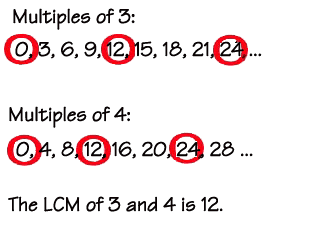## Search Here

### LOWEST COMMON MULTIPLE (L.C.M.).

A common multiple is a number that is a multiple of two or more numbers. The common multiples of 3 and 4 are 0, 12, 24, ....

The Least Common Multiple (LCM) of two numbers is the smallest number (not zero) that is a multiple of both.### LOWEST COMMON MULTIPLE (L.C.M.)

The Lowest Common Multiple (L.C.M)is the smallest number that is a common multiple of two or more numbers.
For example, the L.C.M of 3 and 5 is 15 .(see the example above).

The simple method of finding the L.C.Mof smaller numbers is to write down the multiples of the larger numberuntil one of them is also a multiple of the smaller number.

#### Example :

Find the Lowest Common Multiple of 8 and 12.
Solution: Multiples of 12 are 12, 24...
24 is also a multiple of 8, so the L.C.M of 8 and 12 is 24.